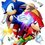# A combinatorics problem..

There are $n$ (distinct) pairs of gloves. In how many ways can $n$ people select a left handed and right handed glove, such that they do not select a pair? Please give me an expression in terms of $n$.Note by Divyaanand Sinha
6 years, 6 months ago

This discussion board is a place to discuss our Daily Challenges and the math and science related to those challenges. Explanations are more than just a solution — they should explain the steps and thinking strategies that you used to obtain the solution. Comments should further the discussion of math and science.

When posting on Brilliant:

• Use the emojis to react to an explanation, whether you're congratulating a job well done , or just really confused .
• Ask specific questions about the challenge or the steps in somebody's explanation. Well-posed questions can add a lot to the discussion, but posting "I don't understand!" doesn't help anyone.
• Try to contribute something new to the discussion, whether it is an extension, generalization or other idea related to the challenge.

MarkdownAppears as
*italics* or _italics_ italics
**bold** or __bold__ bold
- bulleted- list
• bulleted
• list
1. numbered2. list
1. numbered
2. list
Note: you must add a full line of space before and after lists for them to show up correctly
paragraph 1paragraph 2

paragraph 1

paragraph 2

[example link](https://brilliant.org)example link
> This is a quote
This is a quote
    # I indented these lines
# 4 spaces, and now they show
# up as a code block.

print "hello world"
# I indented these lines
# 4 spaces, and now they show
# up as a code block.

print "hello world"
MathAppears as
Remember to wrap math in $$ ... $$ or $ ... $ to ensure proper formatting.
2 \times 3 $2 \times 3$
2^{34} $2^{34}$
a_{i-1} $a_{i-1}$
\frac{2}{3} $\frac{2}{3}$
\sqrt{2} $\sqrt{2}$
\sum_{i=1}^3 $\sum_{i=1}^3$
\sin \theta $\sin \theta$
\boxed{123} $\boxed{123}$

Sort by:

There are $n!$ ways for the people to first select a left-hand glove. Selecting a right hand glove is then equivalent to finding the number of derangements.

- 6 years, 6 months ago

But I think it's n! for lefthands and n! for the rights, in total of (n!)^2, isn' it?

- 6 years, 6 months ago

Except each person must have a left hand glove and a right hand glove that aren't a pair.

- 6 years, 6 months ago

I forgot that fact, thanks Jimmy. So I think it'll be (n!)^2 -n!. Is this the correct answer?

- 6 years, 6 months ago

No, that doesn't make sense. There aren't exactly $n!$ ways for the people to select gloves such that at least one of them has a pair. Like Daniel commented, there are $n!$ ways to select the left-hand gloves, and the number of ways to select the right-hand gloves is the number of derangements of $n$ elements, which is denoted by $!n$. It turns out, if you take $\frac{n!}{e}$ (where $e$ is Euler's number) and round it to the nearest integer, then you get $!n$, for all positive integers $n$.

- 6 years, 6 months ago

Sorry man. I did not study that part of combinatorics yet. Probably I'll see that soon. Thanks for explaning it for me.

- 6 years, 6 months ago

That's okay :) I actually didn't know about the number of derangements before I did research after seeing this question.

- 6 years, 6 months ago

Your question is incomplete. You are not mentioning whether each person takes equal no(2) of gloves or anyone can select any no. of gloves. If there is no constraint on the no. of gloves a person can choose , then ans. is $\binom{n}{2}^2$

- 6 years, 6 months ago

I see that each person selects "a left-handed and a right-handed glove", indicating at least one of each? And by the condition that there are only $n$ pairs of gloves and $n$ people, it's pretty obvious that each person must select one of each. Of course, if the question was edited after you posted your comment then I can't tell.

Besides, even with your interpretation, for $n=2$ it's wrong; there are $2$ ways (a person chooses one left glove and the right glove that doesn't make a pair and the other person takes the rest; there are two such pairs) while your formula gives $1$.

- 6 years, 6 months ago